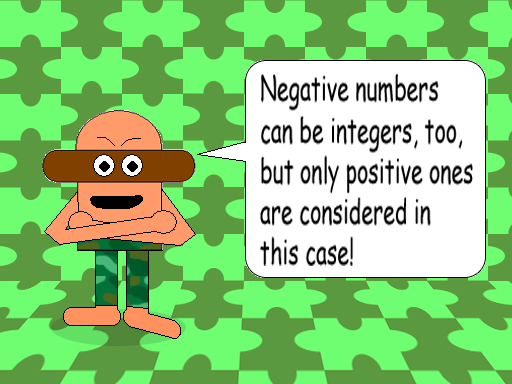# Super Subtraction 2: The Formula for the Difference of Consecutive Integers From 1 to x

## Remember this formula from Math Trick #18?

(x^2 + x)/2 = y

x = the final integer, y = the sum

## Examples:

1 - 2 - 3 - 4 = -8

1 - 2 - 3 - 4 - 5 - 6 = -19

1 - 2 - 3 - 4 - 5 - 6 - 7 - 8 = -34

## Here's the formula for the difference of consecutive integers:

-((x^2 + x)/2) + 2 = y

x = the final integer, y = the difference

Note: The difference is always negative. (If x2)When you see a negative sign to the left of the left parenthesis, it means multiply what's inside by -1.

1 - 2 - 3 - ... - 25 = -323

-((25^2 + 25)/2) + 2 = -((625 + 25)/2) +2 = -(650/2) + 2 = -325 + 2 = -323

In this case, x = 25 & y = -323

If you have a graphing calculator, then you can see that this quadratic function intersects with the original function for sums of consecutive integers! In fact, they intersect twice! (At x = -2 or 1; y = 1 for both intersections)

1 - 2 = -1

-((2^2 + 2)/2) + 2 = -((4 + 2)/2) + 2 = -(6/2) + 2 = -3 + 2 = -1

In this case, x = 2 & y = -1

## This final example is to show why you must multiply the original formula by -1 & then add 2. Surely, you already know that 1 - 1 = 0, but subtracting 2 from 1 gives you a difference that is less than zero & any number less than zero is negative.

Note: Like division, subtraction isn't commutative, either. So the formula of this math trick shows the difference you get if you start at 1.